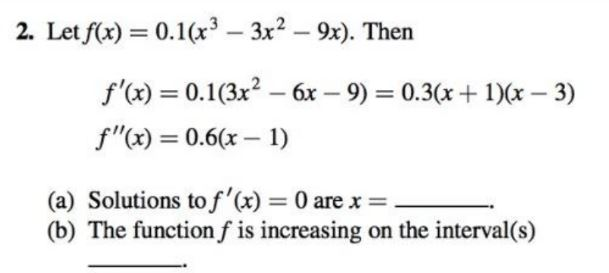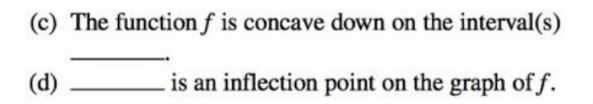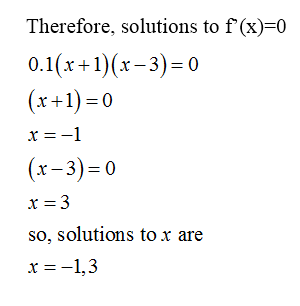# 2. Let f(x) = 0.1(x³ – 3x2 – 9x). Then%3Df'(x) = 0.1(3x? – 6x – 9) = 0.3(x + 1)(x – 3)%3Df"(x) = 0.6(x – 1)%3D(a) Solutions to f'(x) = 0 are x =(b) The function f is increasing on the interval(s)%3D (c) The function f is concave down on the interval(s)is an inflection point on the graph of f.(d)

Question
1 views

with examplehelp_outlineImage Transcriptionclose2. Let f(x) = 0.1(x³ – 3x2 – 9x). Then %3D f'(x) = 0.1(3x? – 6x – 9) = 0.3(x + 1)(x – 3) %3D f"(x) = 0.6(x – 1) %3D (a) Solutions to f'(x) = 0 are x = (b) The function f is increasing on the interval(s) %3D fullscreenhelp_outlineImage Transcriptionclose(c) The function f is concave down on the interval(s) is an inflection point on the graph of f. (d) fullscreen
check_circle

Step 1

Since we are allowed to answer three subparts only and you have not mentioned the subparts is to be done. Hence, the first three subparts are answered here.

Step 2Step 3...

### Want to see the full answer?

See Solution

#### Want to see this answer and more?

Solutions are written by subject experts who are available 24/7. Questions are typically answered within 1 hour.*

See Solution
*Response times may vary by subject and question.
Tagged in

### Calculus